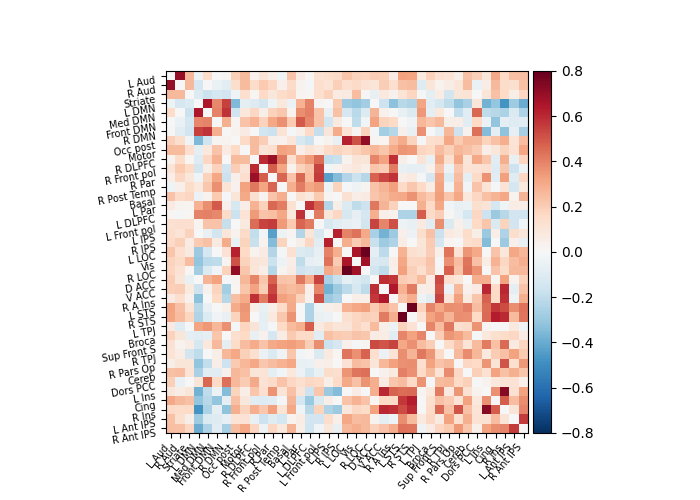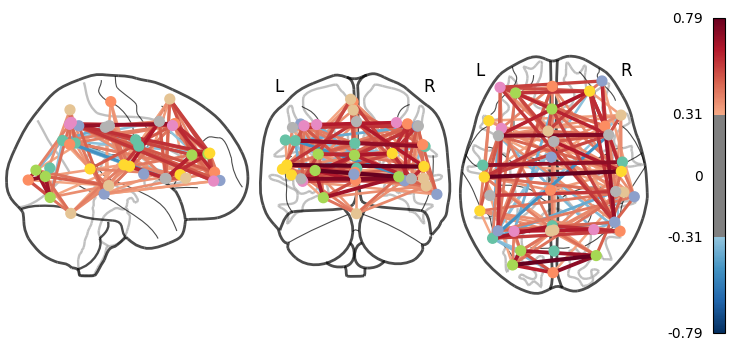# Extracting signals of a probabilistic atlas of functional regions#

This example extracts the signal on regions defined via a probabilistic atlas, to construct a functional connectome.

We use the MSDL atlas of functional regions in movie-watching.

The key to extract signals is to use the `nilearn.maskers.NiftiMapsMasker` that can transform nifti objects to time series using a probabilistic atlas.

As the MSDL atlas comes with (x, y, z) MNI coordinates for the different regions, we can visualize the matrix as a graph of interaction in a brain. To avoid having too dense a graph, we represent only the 20% edges with the highest values.

Note

If you are using Nilearn with a version older than `0.9.0`, then you should either upgrade your version or import maskers from the `input_data` module instead of the `maskers` module.

That is, you should manually replace in the following example all occurrences of:

```from nilearn.maskers import NiftiMasker
```

with:

```from nilearn.input_data import NiftiMasker
```

## Retrieve the atlas and the data#

```from nilearn import datasets

atlas = datasets.fetch_atlas_msdl()
atlas_filename = atlas["maps"]
labels = atlas["labels"]

data = datasets.fetch_development_fmri(n_subjects=1)

print(
"First subject resting-state nifti image (4D) is located "
f"at: {data.func}"
)
```
```First subject resting-state nifti image (4D) is located at: /home/remi/nilearn_data/development_fmri/development_fmri/sub-pixar123_task-pixar_space-MNI152NLin2009cAsym_desc-preproc_bold.nii.gz
```

## Extract the time series#

```from nilearn.maskers import NiftiMapsMasker

maps_img=atlas_filename,
standardize="zscore_sample",
standardize_confounds="zscore_sample",
memory="nilearn_cache",
verbose=5,
)
```
```[NiftiMapsMasker.fit] loading regions from None
Resampling maps
/home/remi/github/nilearn/env/lib/python3.11/site-packages/nilearn/_utils/cache_mixin.py:241: UserWarning:

memory_level is currently set to 0 but a Memory object has been provided. Setting memory_level to 1.

________________________________________resample_img cache loaded - 0.1s, 0.0min
__________________________________filter_and_extract cache loaded - 0.0s, 0.0min
```

We can generate an HTML report and visualize the components of the `NiftiMapsMasker`. You can pass the indices of the spatial maps you want to include in the report in the order you want them to appear. Here, we only include maps 2, 6, 7, 16, and 21 in the report:

```report = masker.generate_report(displayed_maps=[2, 6, 7, 16, 21])
report
```
```/home/remi/github/nilearn/env/lib/python3.11/site-packages/numpy/ma/core.py:2820: UserWarning:

Warning: converting a masked element to nan.
```

# NiftiMapsMasker Class for masking of Niimg-like objects. NiftiMapsMasker is useful when data from overlapping volumes should be extracted (contrarily to :class:`nilearn.maskers.NiftiLabelsMasker`). Use case: Summarize brain signals from large-scale networks obtained by prior PCA or :term:`ICA`. Note that, Inf or NaN present in the given input images are automatically put to zero rather than considered as missing data.This reports shows the spatial maps provided to the mask.

The masker has 39 spatial maps in total.

Only 5 spatial maps are shown in this report.

Parameter Value
allow_overlap True
detrend False
dtype None
high_pass None
high_variance_confounds False
low_pass None
maps_img /home/remi/nilearn_data/msdl_atlas/MSDL_rois/msdl_rois.nii
memory Memory(location=nilearn_cache/joblib)
memory_level 1
reports True
resampling_target data
smoothing_fwhm None
standardize zscore_sample
standardize_confounds zscore_sample
t_r None
verbose 5

time_series is now a 2D matrix, of shape (number of time points x number of regions)

```print(time_series.shape)
```
```(168, 39)
```

## Build and display a correlation matrix#

```from nilearn.connectome import ConnectivityMeasure

correlation_measure = ConnectivityMeasure(
kind="correlation",
standardize="zscore_sample",
)
correlation_matrix = correlation_measure.fit_transform([time_series])

# Display the correlation matrix
import numpy as np

from nilearn import plotting

# Mask out the major diagonal
np.fill_diagonal(correlation_matrix, 0)
plotting.plot_matrix(
correlation_matrix, labels=labels, colorbar=True, vmax=0.8, vmin=-0.8
)
``````<matplotlib.image.AxesImage object at 0x7fd1f29dacd0>
```

## And now display the corresponding graph#

```from nilearn import plotting

coords = atlas.region_coords

# We threshold to keep only the 20% of edges with the highest value
# because the graph is very dense
plotting.plot_connectome(
correlation_matrix, coords, edge_threshold="80%", colorbar=True
)

plotting.show()
```## 3D visualization in a web browser#

An alternative to `nilearn.plotting.plot_connectome` is to use `nilearn.plotting.view_connectome` that gives more interactive visualizations in a web browser. See 3D Plots of connectomes for more details.

```view = plotting.view_connectome(
correlation_matrix, coords, edge_threshold="80%"
)

# In a Jupyter notebook, if ``view`` is the output of a cell, it will
# be displayed below the cell
view
```